# A mass of 65.0 g is attached to the spring. A spring ( = 1700 N/m)...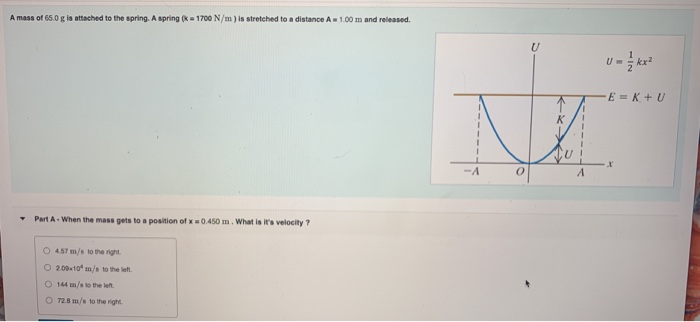A mass of 65.0 g is attached to the spring. A spring ( = 1700 N/m) is stretched to a distance A - 1.00 m and released U kx E = K + U K Part A. When the mass gets to a position of x 0.450 m. What is it's velocity? O 457 m/s to the night 200x109 m/s to the O 144 m/s to the left 72.8 m/s to the right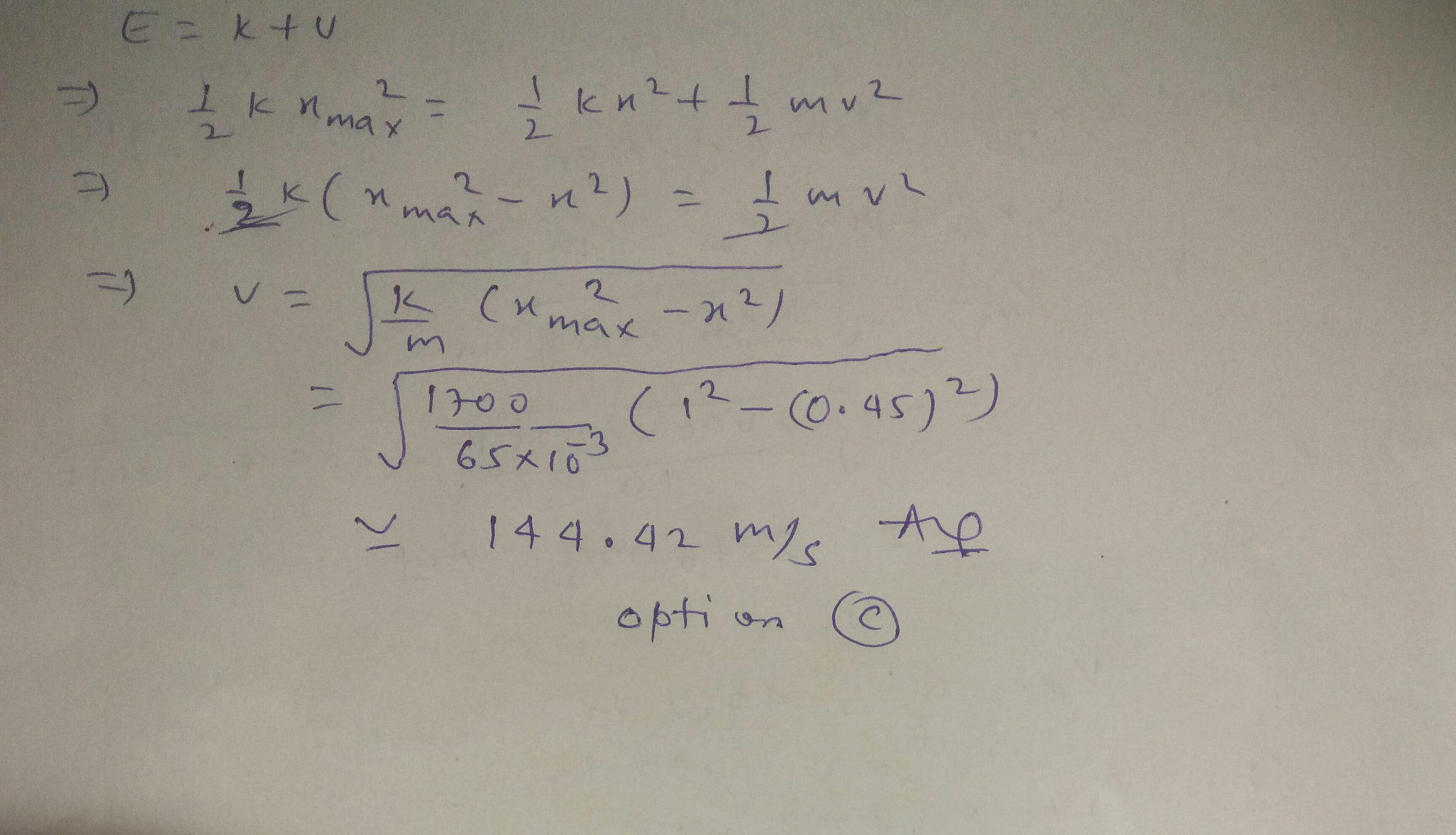#### Earn Coin

Coins can be redeemed for fabulous gifts.

Similar Homework Help Questions
• ### A 2.1 kg mass is attached to a spring with a spring constant of 75.3 N/m....

A 2.1 kg mass is attached to a spring with a spring constant of 75.3 N/m. The spring is securely attached on the other end to a vertical wall so that the spring extends and contracts horizontally. The mass is shoved so the mass has a velocity of 3.1 m/s and the spring is streched 0.128 m from its equilibrium, or rest, position when the mass is released. Friction and air resistance are negligible. What is the greatest distance that...

• ### A spring of spring constant k=261 N/m is attached to a block of mass 1.38 kg...

A spring of spring constant k=261 N/m is attached to a block of mass 1.38 kg and stretched horizontally to a position 15.0 cm from the springs equilibrium position. The spring and mass are released and oscillate in simple harmonic motion across a frictionless horizontal surface. What is the maximum speed obtained by the mass? m/s

• ### Ignore damping forces. A mass of 4 kg is attached to a spring with constant k- 16 N/m, then the s...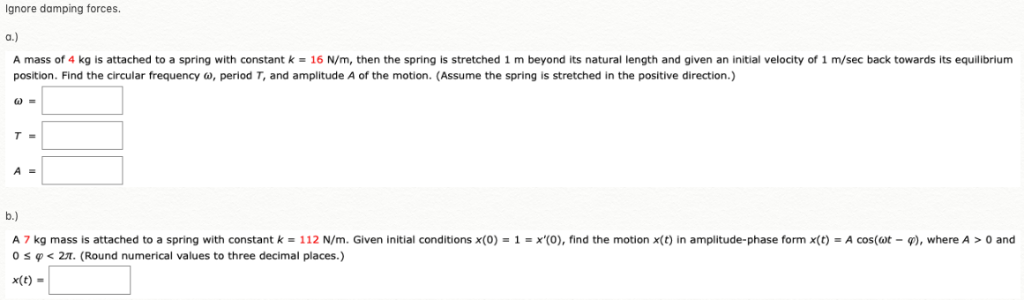Ignore damping forces. A mass of 4 kg is attached to a spring with constant k- 16 N/m, then the spring is stretched 1 m beyond its natural length and given an initial velocity of 1 m/sec back towards its equilibrium position. Find the circular frequency ω, period T, and amplitude A of the motion. (Assume the spring is stretched in the positive direction.) A 7 kg mass is attached to a spring with constant k 112 N m. Given...

• ### A block of mass m= 10.0kg is attached to the end of an ideal spring. Due...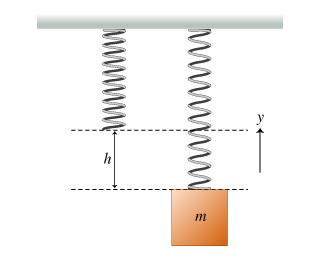A block of mass m= 10.0kg is attached to the end of an ideal spring. Due to the weight of the block, the block remains at rest when the spring is stretched a distance h= 8.00cm from its equilibrium length. (Figure 1) The spring has an unknown spring constant k. Take the acceleration due to gravity to be g = 9.81m/s2 . What is the spring constant k? Express your answer in newtons per meter.         k...

• ### A block of mass m = 6.04 kg is attached to a spring with spring constant...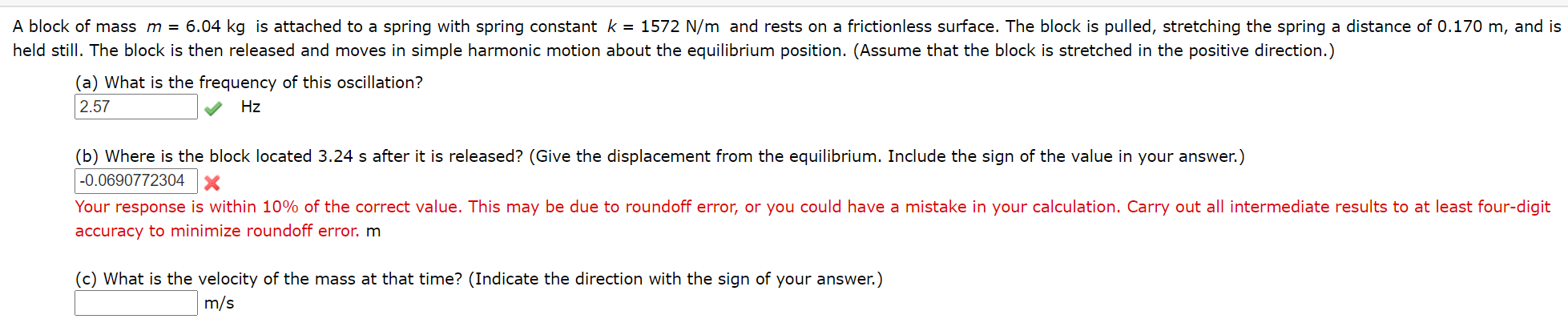A block of mass m = 6.04 kg is attached to a spring with spring constant k = 1572 N/m and rests on a frictionless surface. The block is pulled, stretching the spring a distance of 0.170 m, and is held still. The block is then released and moves in simple harmonic motion about the equilibrium position. (Assume that the block is stretched in the positive direction.) (a) What is the frequency of this oscillation? 2.57 Hz (b) Where is...

• ### (16 pts) A mass m is attached to a spring which is held stretched a distance...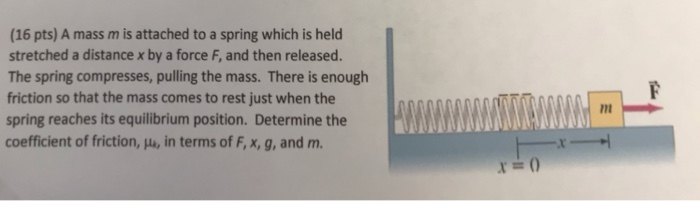(16 pts) A mass m is attached to a spring which is held stretched a distance x by a force F, and then released. The spring compresses, pulling the mass. There is enough riction so that the mass comes to rest just when the spring reaches its equilibrium position. Determine the coefficient of friction, μ, in terms of FMA and m.

• ### 9. A mass m is attached to a massless spring with a force constant k. The...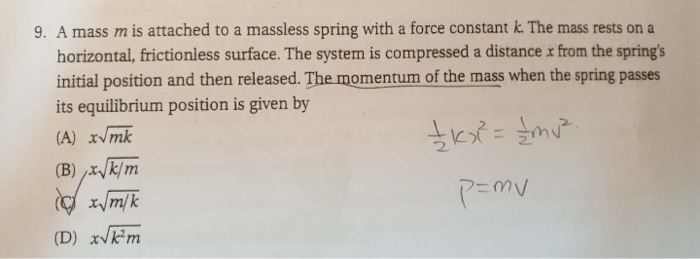9. A mass m is attached to a massless spring with a force constant k. The mass rests on a horizontal, frictionless surface. The system is compressed a distance x from the spring's initial position and then released. The momentum of the mass when the spring passes its equilibrium position is given by (A) xvmek (B) x/k/m o x/m/k (D) x/km + KxP = {mv² p=mv

• ### 13.7 A 5 kg mass is attached to a spring with a spring constant of 490...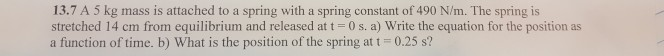13.7 A 5 kg mass is attached to a spring with a spring constant of 490 N/m. The spring is stretched 14 cm from equilibrium and released at t = 0 s. a) Write the equation for the position as a function of time. b) What is the position of the spring at t 0.25 s?

• ### 2. A spring with constant 1.46 N/m has an unknown mass attached to it. It is...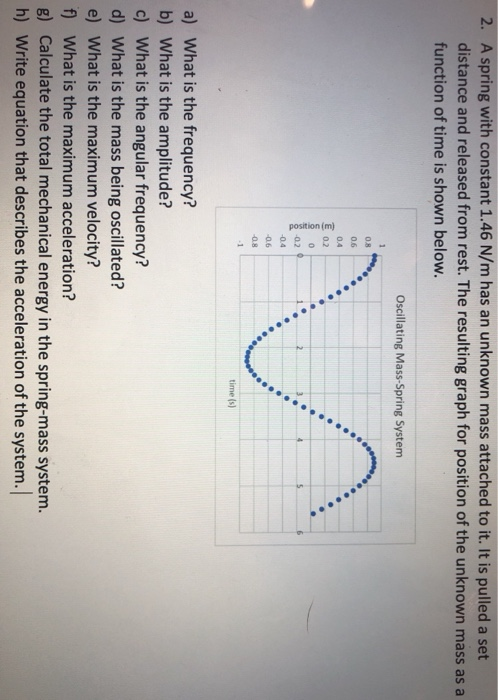2. A spring with constant 1.46 N/m has an unknown mass attached to it. It is pulled a set distance and released from rest. The resulting graph for position of the unknown mass as a function of time is shown below. Oscillating Mass-Spring System 08 0.6 0.4 02 position (m) 0 -02 5 -0.6 -0.8 1 time (s) a) What is the frequency? b) What is the amplitude? c) What is the angular frequency? d) What is the mass being...

• ### A small box with mass 0.6 kg ﻿ is attached to a spring (k=250 N/m )...

A small box with mass 0.6 kg ﻿ is attached to a spring (k=250 N/m ) and oscillates left and right. At a particular moment, the box is 20 cm to the right of its equilibrium position moving left with a speed of 4 m/s . a) What is the maximum distance to the right of its equilibrium position the box will reach? (cm) b) What is the maximum speed the box will have? (m/s) c) What is the period...